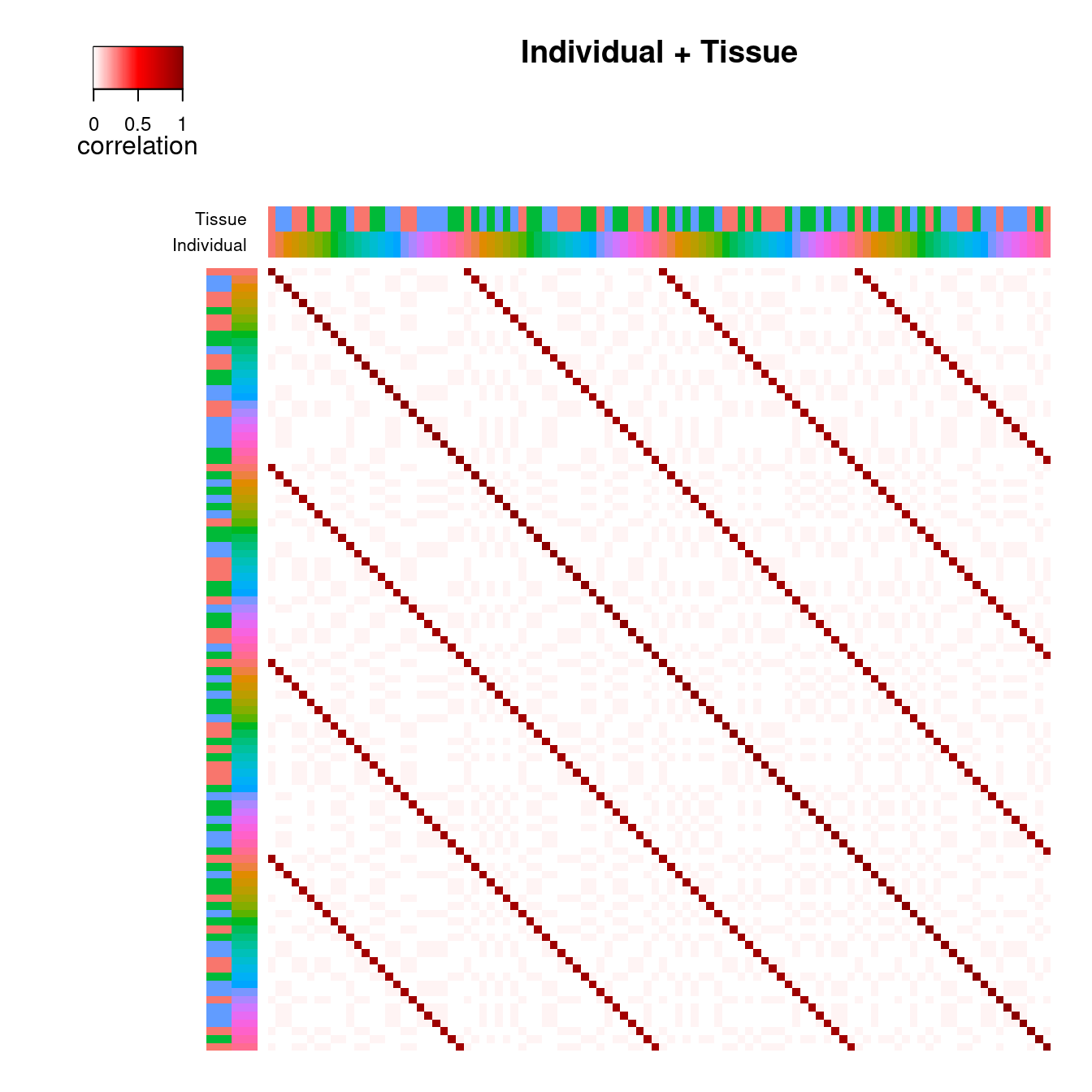The correlation structure between samples in complex study designs can be decomposed into the contribution of multiple dimensions of variation. variancePartition provides a statistical and visualization framework to interpret sources of variation. Here I describe a visualization of the correlation structure between samples for a single gene.

In the example dataset described in the main vignette, samples are correlated because they can come from the same individual or the same tissue. The function plotCorrStructure() shows the correlation structure caused by each variable as well and the joint correlation structure. Figure 1 shows the correlation between samples from the same individual where (a) shows the samples sorted based on clustering of the correlation matrix and (b) shows the original order. Figure 1 c) and d) shows the same type of plot except demonstrating the effect of tissue. The total correlation structure from summing individual and tissue correlation matricies is shown in Figure 2. The code to generate these plots is shown below.

# Plot variance structure

# Fit linear mixed model and examine correlation stucture
# for one gene
data(varPartData)

form <- ~ Age + (1|Individual) + (1|Tissue)

fitList <- fitVarPartModel( geneExpr[1:2,], form, info )

# focus on one gene
fit = fitList[]

## By Individual

### Reorder samples

# Figure 1a
# correlation structure based on similarity within Individual
# reorder samples based on clustering
plotCorrStructure( fit, "Individual" )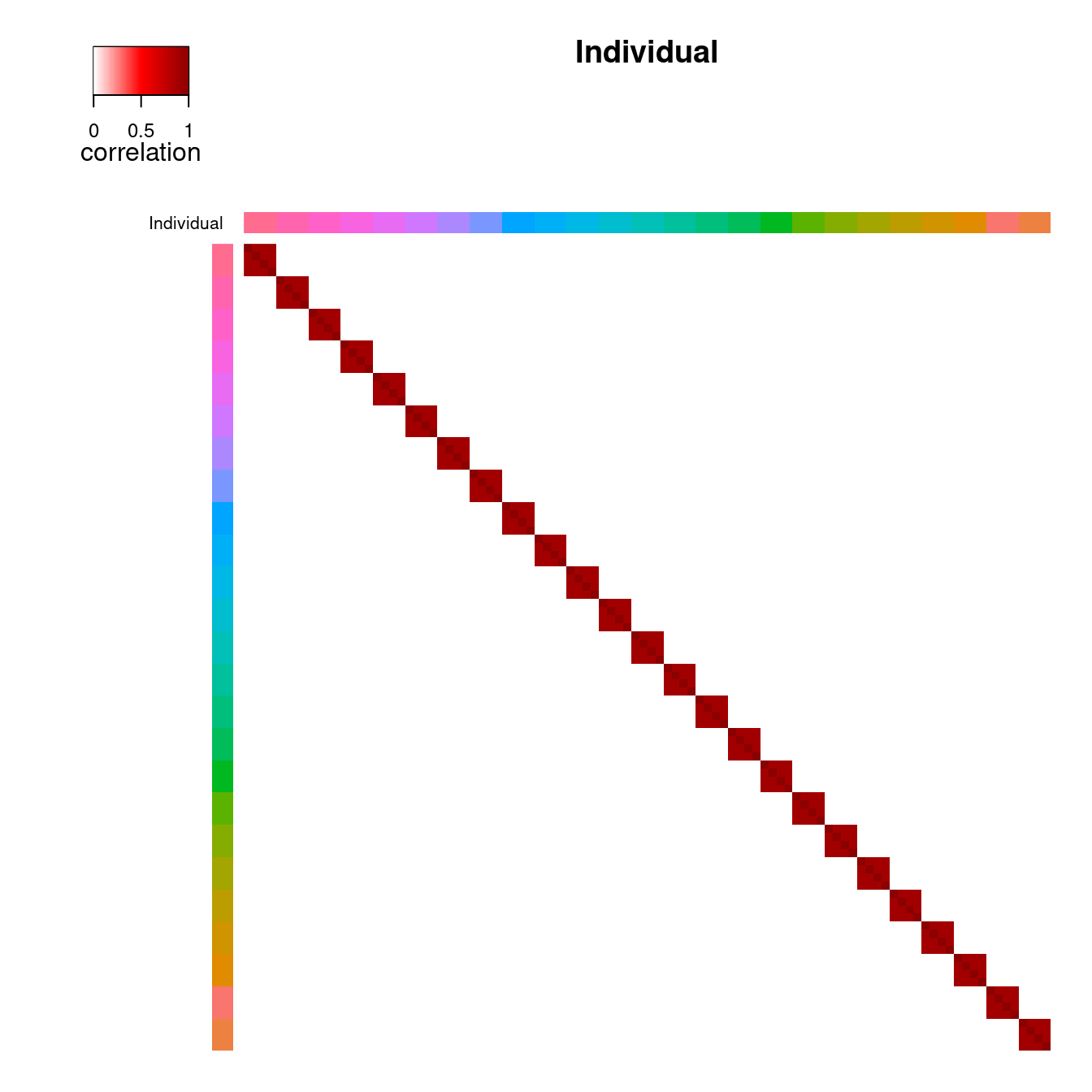### Original order of samples

# Figure 1b
# use original order of samples
plotCorrStructure( fit, "Individual", reorder=FALSE )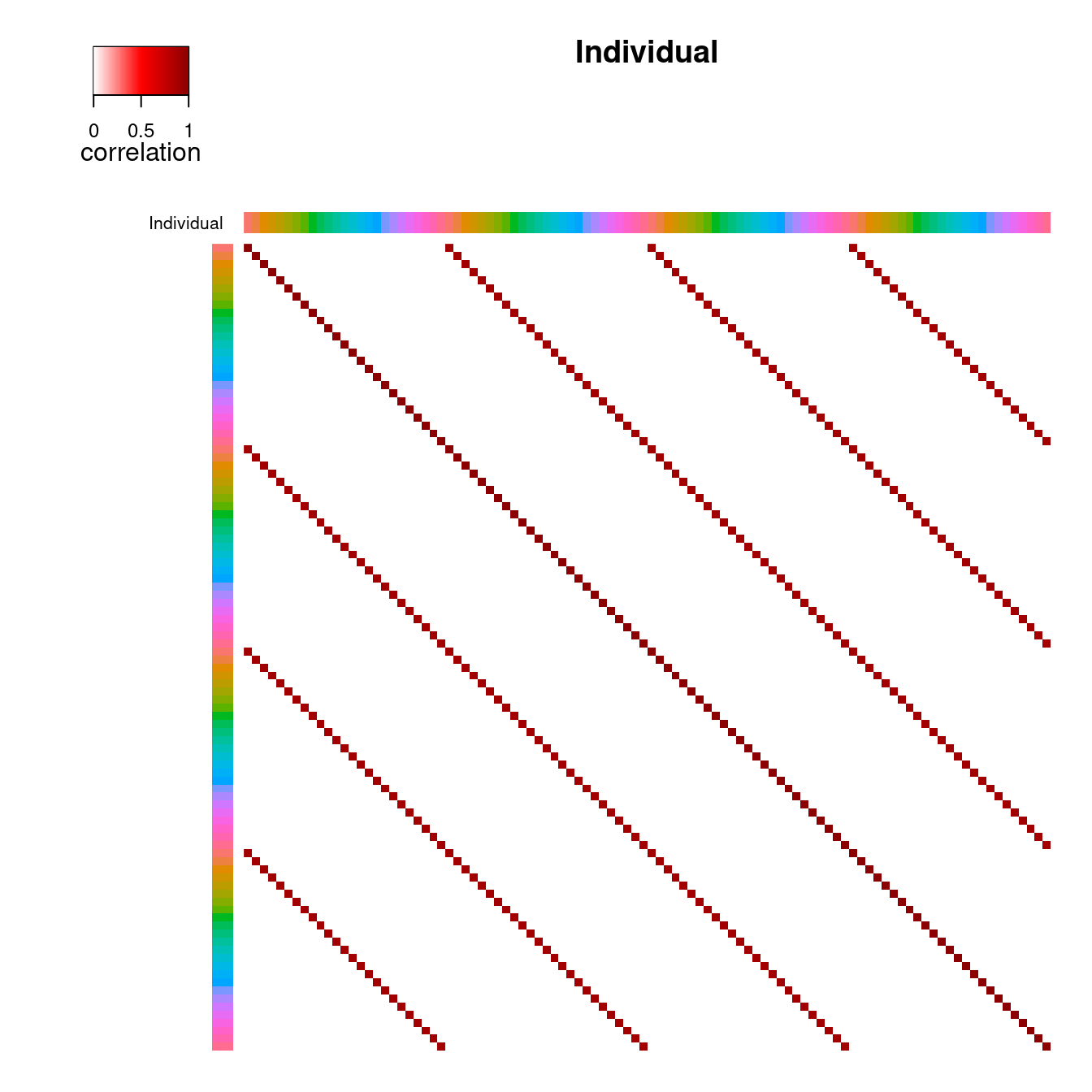## By Tissue

### Reorder samples

# Figure 1c
# correlation structure based on similarity within Tissue
# reorder samples based on clustering
plotCorrStructure( fit, "Tissue" )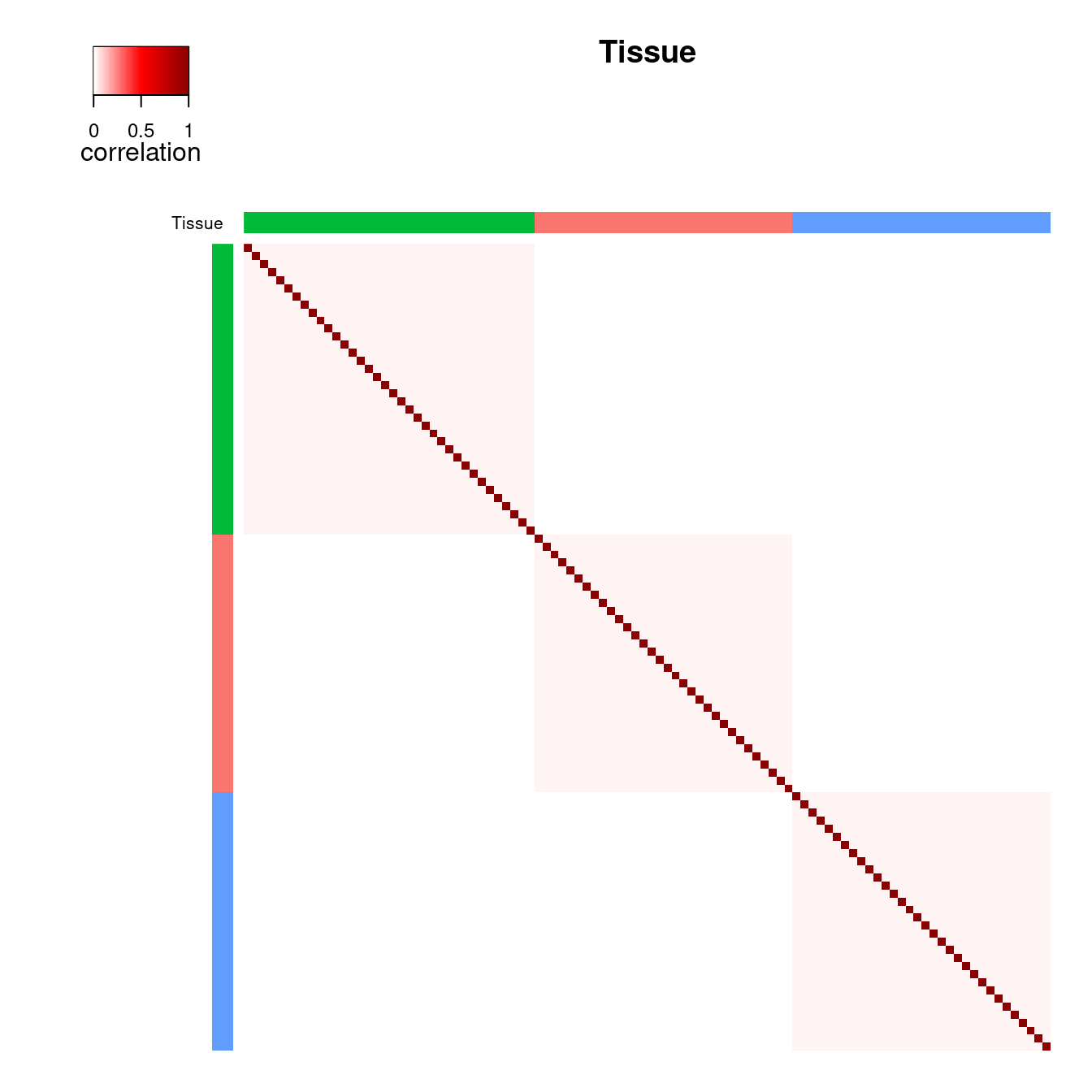### Original order of samples

# Figure 1d
# use original order of samples
plotCorrStructure( fit, "Tissue", reorder=FALSE )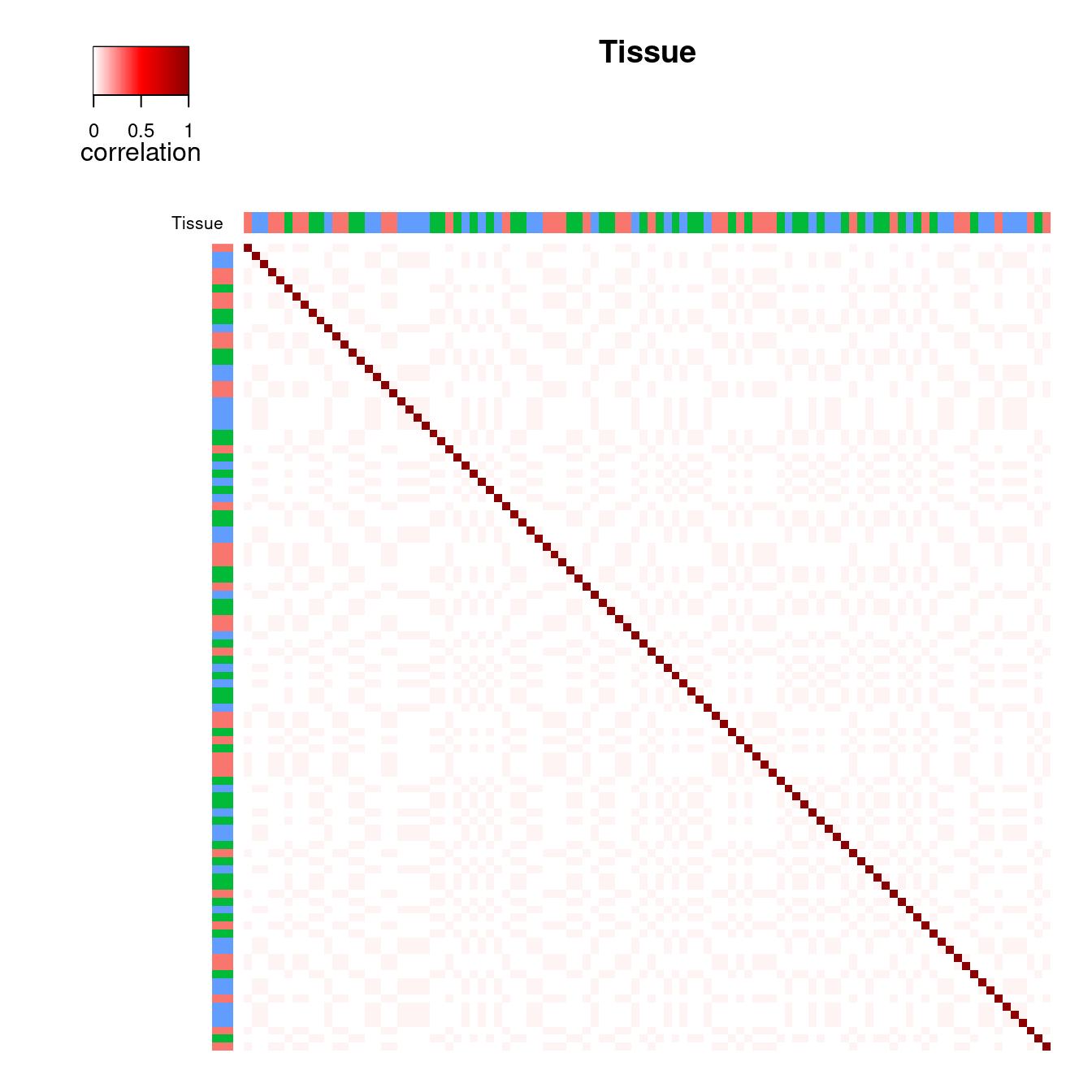## By Individual and Tissue

### Reorder samples

# Figure 2a
# correlation structure based on similarity within
# Individual *and* Tissue, reorder samples based on clustering
plotCorrStructure( fit )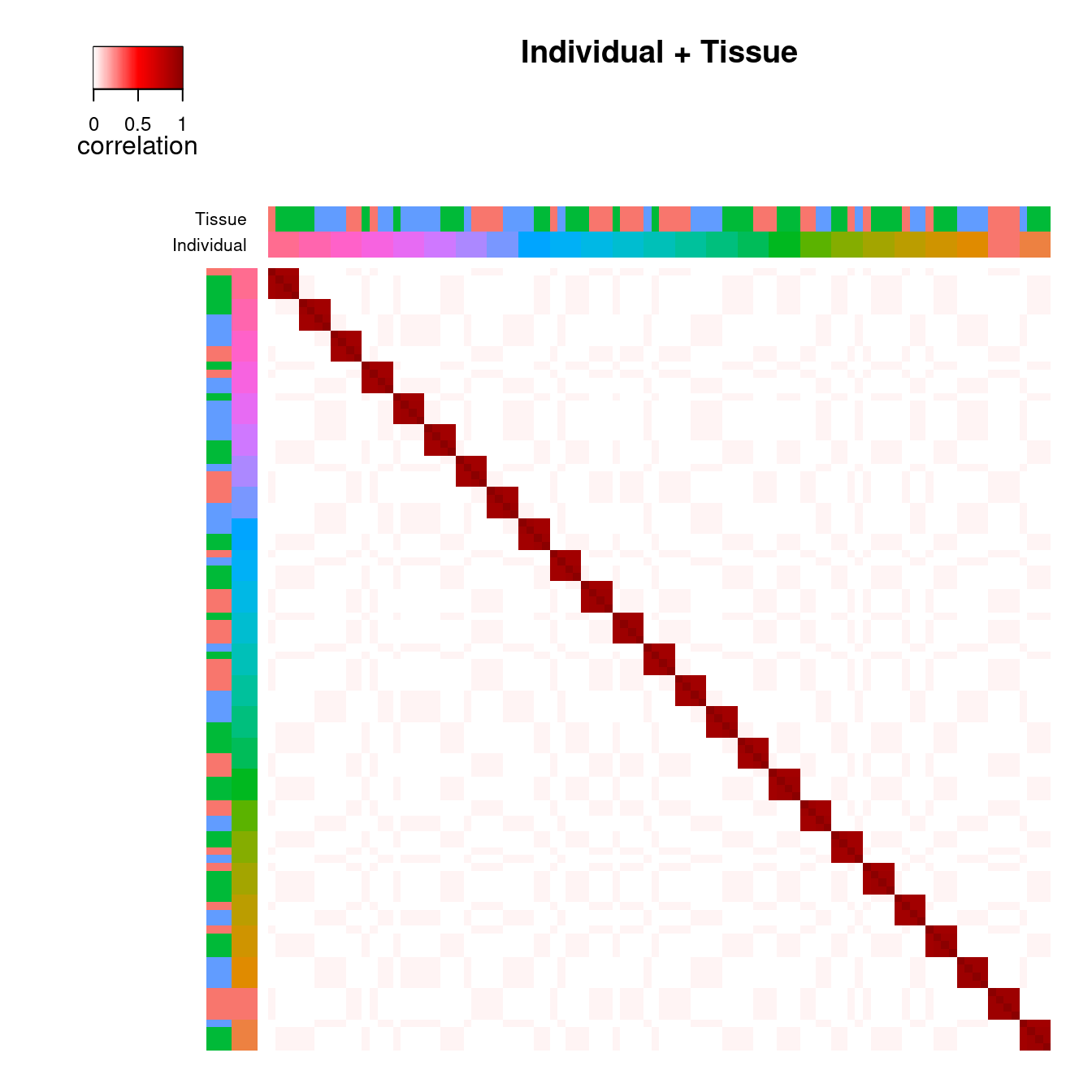### Original order of samples

# Figure 2b
# use original order of samples
plotCorrStructure( fit, reorder=FALSE )